# 欲善其事，先利其器-数组类库 | 带你学《Java面向对象编程》之二十

【本节目标】

## 数组相关操作方法

1. 数组排序：java.util.Arrays.sort(数组名称)。

class ArrayUtil {
public static void printArray(int temp []) {
for (int x = 0 ; x < temp.lenght ; x ++) {
System.out.print (temp[x] + “、”) ;
}
System.out.println() ;
}
}
public class ArrayDemo {
public static void main (String args[ ]) {
int data [] = new int [] {23, 12, 1, 234, 2, 6, 12, 34, 56} ;
java.util.Arrays.sort(data) ;         //排序
ArrayUtil.printArray(data) ;
}
}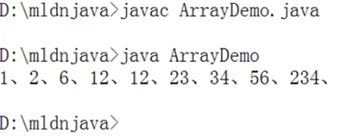1. 数组拷贝（把方法做了一些变形）：
• System.arraycopy(源数组，源数组开始点，目标数组，目标数组开始点，拷贝长度)。

• 现在假设有两个数组：

• 要求拷贝之后的数组二内容为：11、22、33、6、7、8、77、88、99；
class ArrayUtil {
public static void printArray(int temp []) {
for (int x = 0 ; x < temp.lenght ; x ++) {
System.out.print (temp[x] + “、”) ;
}
System.out.println() ;
}
}
public class ArrayDemo {
public static void main (String args[ ]) {
int dataA [] = new int [] {1,2,3,4,5,6,7,8,9} ;
int dataB [] = new int [] {11,22,33,44,55,66,77,88,99} ;
System.arraycopy (dataA,5,dataB,3,3) ;
ArrayUtil.printArray(dataB) ;
}
}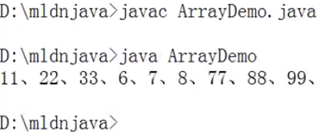class ArrayUtil {
public static void printArray(int temp []) {
for (int x = 0 ; x < temp.lenght ; x ++) {
System.out.print (temp[x] + “、”) ;
}
System.out.println() ;
}
public static void arraycopy(int [] src,int sindex, int dsc[] ,int dindex, int len) {
for(int x = 0 ;x < len ; x ++) {
dsc[dindex ++] = src[sindex ++] ;
}
}
}
public class ArrayDemo {
public static void main (String args[ ]) {
int dataA [] = new int [] {1,2,3,4,5,6,7,8,9} ;
int dataB [] = new int [] {11,22,33,44,55,66,77,88,99} ;
ArrayUtil.arraycopy (dataA,5,dataB,3,3) ;
ArrayUtil.printArray(dataB) ;
}
}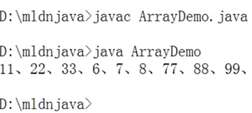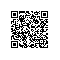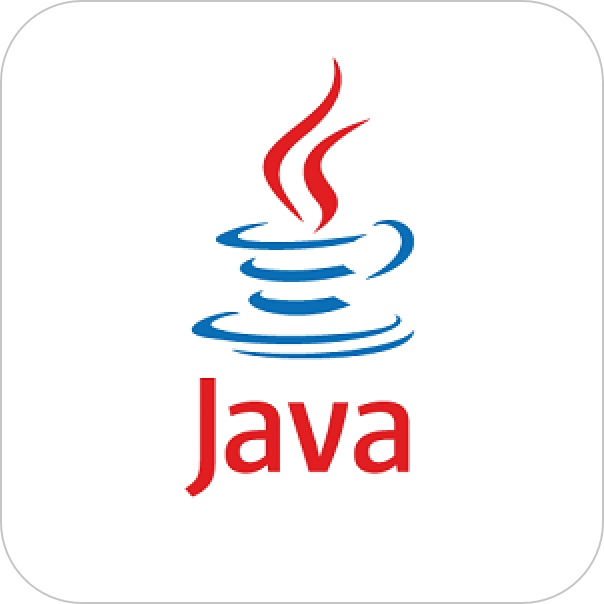Java开发者使用钉钉扫一扫加入圈子
+ 订阅

Java开发者成长课堂，课程资料学习，实战案例解析，Java工程师必备词汇等你来~